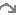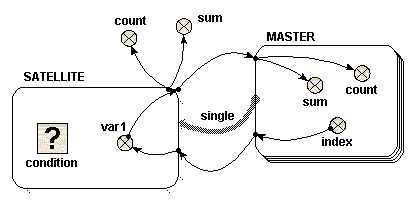#Single role arrow

Using a single role arrow to connect two submodels is almost exactly equivalent to nesting one submodel inside the other. In effect, the submodel that the role arrow points to, is nested inside the submodel that the role arrow points from. This alternative diagram form is useful because of the extra flexibility it allows in laying out the model diagram. It is also helpful to understand this simple use of role arrows before using them in more complex ways.

The following model diagram illustrates the relevant features of the use of a single role arrow to connect two submodels. In this case, one submodel is called "Base" (or "Master"), and the role arrow points from it, to the other submodel, called "Satellite". "Base" is a multiple-instance submodel, with fixed dimensions. In the following example, there are ten instances of "Base". Inside "Base" there is a variable called "index". The equation for "index" is "index(1)". The variable "index" therefore has a unique value within each instance of "Base".When adding the equations for this model, you will notice that the role arrow is having an effect on the parameter names corresponding to influences that come from inside a submodel at its far end. First, have a look at "var1" in the "Satellite" model. Now, if the role arrow were not present, the only available parameter name for this would be "[index]", representing an array of the values of "index" in the 10 instances of "Master". However, with the role arrow present, you can use the parameter name "single_index", made by combining the names of the role arrow and source component. This is a scalar quantity, and has the value of "index" in the particular instance of "Master" associated with each instance of "Sattelite". It is as if "Satellite" were a simple submodel inside "Master", with one instance for each instance of its parent. (Note, if using Simile v5.7 or later, the parameter name "[every_index]" is also available, which stands for the array of 10 values that you would get if the role arrow were not present. In versions 6 and on, the availability of the different interpretations of an influence can be enabled or disabled in the influence properties dialogue -- see Influences and Roles).

Something similar happens for influences going in the other direction from the role arrow. Now, look at "sum" in the "Master" model. The parameter name "{var1_single}" is available. The curly brackets indicate that this stands for a list of values -- but because the role arrow is used, the list will contain only the values from instances of "Satellite" associated with each particular instance of "Master". In this case, that means at most one instance, and hence one value, for each instance of the base model. (Again, if using Simile v5.7 or later, the parameter name "{every_var1}" is also available, which stands for the list containing values from all instances of the associated model).

Now let's look at what happens on execution. To start with, consider a case in which thecondition symbol in "Satellite" is omitted (or equivalently, where it has an equation whose value is always "true", such as 1>0). In this case, ten instances of "Satellite" are created, the same as the number of instances of "Base". Inside "Satellite" is a variable called "var1". There is an influence arrow from the variable "index" in "Base" to "var1", and its equation is simply "single_index". The value of "var1" in each instance of Satellite is therefore equal to the "index" of the equivalent instance of "Base".

To illustrate how "var1" is treated, there are four influence arrows taken from it. Two influence arrows point to variables "count" and "sum" within "Base" and two point to variables "count" and "sum" outside "Base". There is an important difference in the values received in these two circumstances, which is easy to understand if you consider "Satellite" is treated as if it were nested inside "Base".

• Inside "Base", the equation "count({var1_single})" returns 1, and "sum({var1_single})" returns a value equal to "index" of the same instance.
• Outside "Base", the equation "count({var1})" returns 10, and "sum({var1})" returns a value equal to sum of the index of all the instances, i.e. 55, in this case (calculated from 1+2+3+…+9+10)

Beware one point: both inside and outside "Base", the value returned from "Satellite" is received as a list, even if there is no condition symbol in the satellite. An integrating function such as sum() or count() must be used immediately on a list. Inside "Base", the list consists of a single number, so sum({var1_single}) returns the value of the number. The reason for using a list is that the list may in fact be empty, rather than containing a single value, for reasons described below. Note that curly braces are used to denote the list.

Now consider a simple equation for the condition symbol. In the example given, the condition symbol has no influence arrows pointing to it, though it can have. Suppose one wishes "Satellite" to exist for only the odd-numbered instances of "Base". In this case, the equation "fmod(index(1),2)==1" or "index(1)%2==1" would be used. Note that the index() function used within "Satellite" refers to the index of the equivalent instance of "Base".

Using the condition symbol in this or other ways permits the number of instances of "Satellite" to be less than the number of instances of "Base". There can never be more instances of "Satellite" however than instances of "Base", unless the satellite submodel has dimensions of its own.

In the example chosen above, in which only odd-numbered instances of "Satellite" are created, the four influence arrows behave like this.

• Inside "Base", the equation "count({var1_single})" returns either zero or one. It returns one, only if this is an odd-numbered instance, i.e. for instances 1, 3, 5, 7 and 9. Otherwise it returns zero. In these cases, i.e. instances, 2, 4, 6, 8 and 10, the list is an empty list. Similarly, the equation "sum({var_single})" returns either zero or a value equal to "index" of the same instance. It returns zero for each empty list, i.e. instances 2, 4 ,6, 8 and 10.
• Outside "Base", the equation "count({var1})" returns five. The equation "sum({var1})" returns 25 (calculated from 1+3+5+7+9).

When using the condition symbol in "Satellite", the use of the role arrow is more convenient than the equivalent nested arrangement. If "Satellite" were nested inside "Base" it would not be possible directly to receive the list in a variable outside "Base" -- you would instead get an array of lists.

## Many satellite instances per base instance

In the above example, the satellite submodel does not have any dimensions of its own. It only has multiple instances because the base model does, and each base model instance can have at most one associated satellite submodel instance. But you can set dimensions for the satellite model, in which case each base model instance can be associated with up to this number of satellite model instances.

If you do this, then for equations inside the satellite submodel, index(1) means the index of that instance within the group associated with its base model instance, while index(2) means the index of the base model instance (as long as the satellite submodel has only one dimension).In other words, the value that is index(1) in a base model index is available as index(2) in its satellite model instances.

As an example, you could create a model where base instance 1 has 1 satellite instance, base instance 2 has 2, and so forth. The satellite would have dimensions 10 and the condition equation would be "index(1) <= index(2)". In the satellite, "var1" still has the index value of the corresponding base model. There would then be 1+2+...+10 == 55 satellite instances, and the value of "sum" outside the submodels would be 1*1 + 2*2 + ... + 10*10 = 385. Another example: set the condition equation to "index(1) >= index(2)". Now, base instance 1 will have 10 satellite instances, instance 2 has 9 and so forth. There are still 55 satellite instances, but "sum" is now 1*10 + 2*9 + ... + 10*1 = 220.